Sound Design - How To Make An Electric Piano Using Native-Instruments Massive | The GM Community# Sound DesignHow To Make An Electric Piano Using Native-Instruments Massive

This sound design tutorial shows how to make a basic electric piano using Native-Instruments Massive VST wavetable Synthesizer.

1) Initialize Massive VST to its default preset.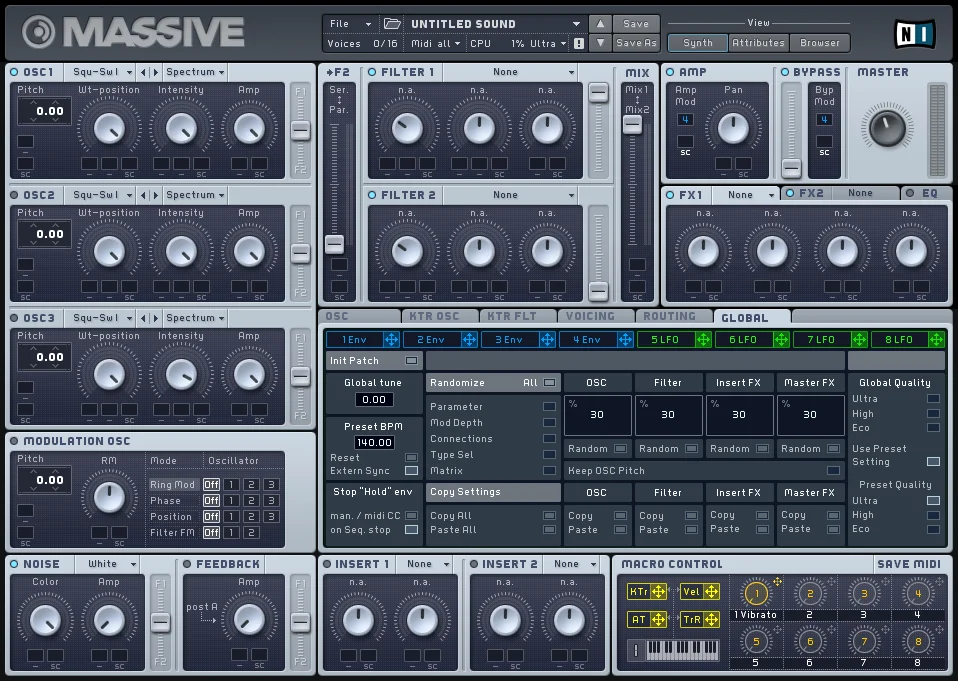2) For Oscillator 1 choose a "Sin-Squ" wavetable, and turn the wavetable position knob completely to the left.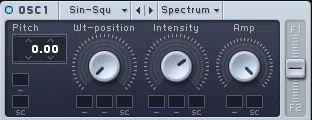3) For Oscillator 2 use "Sin-Squ" wavetable and apply the following settings:
• Pitch = +1 Octave
• Intensity = 70%
• Amp = 80%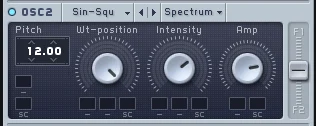4) For Filter 1 apply the following settings:
• Filter Type = Lowpass 4
• Cutoff = 37%
• Resonance = 0%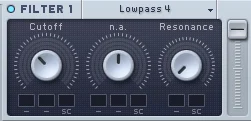5) Clear the Vibrato macro mod assignments and make sure you enable "restart via gate" on the 'Oscillator Tab'.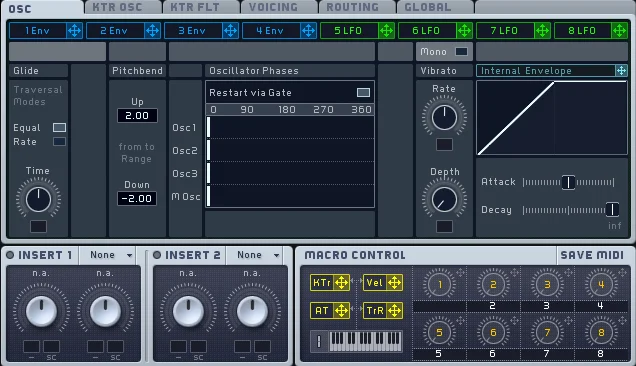6) Now let's move on to the 'AMP Envelope' and change the settings with the following adjustments:
• Attack = 0%
• Decay = 60%
• Decay Level = 40%
• Release = 20%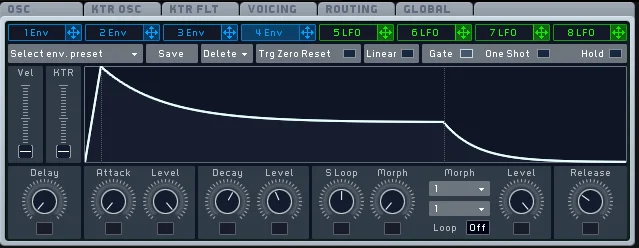7) Tweak the voicing section of Native Instruments Massive like so:
• Unisono = 1
• Play Mode = Polyphonic
• Trigger = Always
• Pitch Cutoff = 7%
• Pan Position = 37%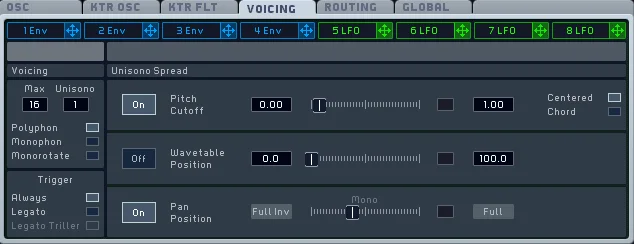8) Click on the routing tab and change the routing for Insert 1 to come before the Filter.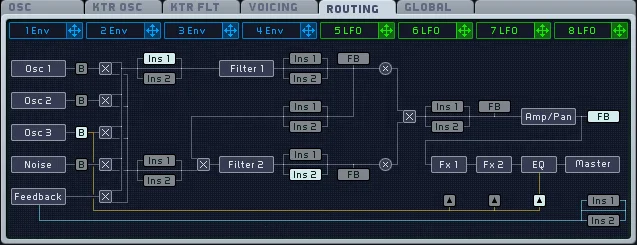9) For an insert effect let's use a "Sine Shaper" with the following configurations:
• Dry/Wet = 30%
• Drive = 50%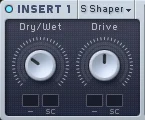10) Let's adjust the parameters of the LFO with the following settings:
• Ratio = 1/8
• LFO Amp = 30%
• Xfade Curve = 1 (i.e all the way up)
• LFO Curve Select = Sine Wave
• LFO Sync = Enabled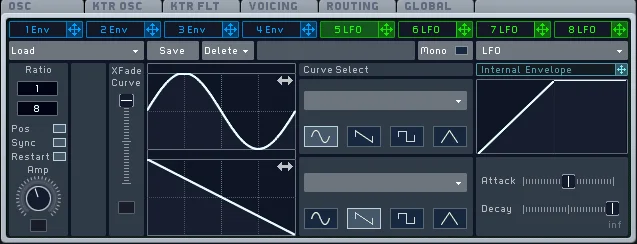11) Let's map LFO 1 to "Amp Level" of both oscillators (i.e OSC 1 & OSC 2). This will create a little bit of a tremolo effect.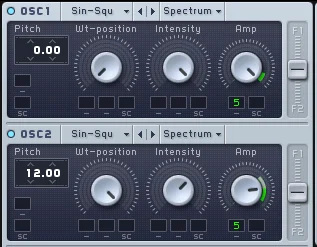12) Adjust the 'Master Output Level' with a value of around 65% in order to compensate for any loss in amplitude.13) Add a "synced delay effect" with the following settings.
• Dry/Wet = 15%
• Damp = 65%
• Feedback = 50%
• Left = 1/4
• Right = 2/8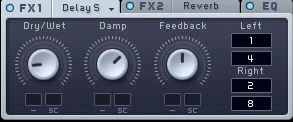14) Add a "reverb effect" with the following settings:
• Dry/Wet = 5%
• Size = 22%
• Density = 60%
• Color = 20%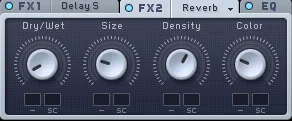You May Also Like:
Author
Goldmidi
First release
Last update
Rating
0 ratings